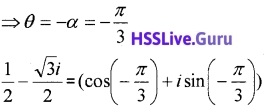# Plus One Maths Chapter Wise Questions and Answers Chapter 5 Complex Numbers and Quadratic Equations

Students can Download Chapter 5 Complex Numbers and Quadratic Equations Questions and Answers, Plus One Maths Chapter Wise Questions and Answers helps you to revise the complete Kerala State Syllabus and score more marks in your examinations.

## Kerala Plus One Maths Chapter Wise Questions and Answers Chapter 5 Complex Numbers and Quadratic Equations

### Plus One Maths Complex Numbers and Quadratic Equations Three Mark Questions and Answers

Plus One Maths Chapter Wise Questions And Answers Pdf Chapter 5 Question 1.
If z1 = 2 – i, z2 = 1 + i

1. Find | z1 + z2 + 1| and |z1 – z2 + i| (2)
2. Hence find $$\left|\frac{z_{1}+z_{2}+1}{z_{1}-z_{2}+i}\right|$$ (1)

1. |z1 + z2 + 1| = |2 – i + 1 + i + 1| = 4
|z1 – z2 + i| = |2 – i – 1 – i + i| = |1 – i|
$$=\sqrt{1+1}=\sqrt{2}$$

2.Hsslive Maths Textbook Answers Plus One Chapter 5 Question 2.
Find the square root of -15 – 8i.
Let x + iy = $$\sqrt{-15-8 i}$$
Then (x + iy)2 = -15 – 8i
⇒ x2 – y2 + 2xyi = – 15 – 8i
Equating real and imaginary parts, we have
x2 – y2 = -15 ______(1)
2xy = – 8
We know the identity
(x2 + y2)2 = (x2 – y2)2 + (2xy)2
= 225 + 64
= 289
Thus, x2 + y2 = 17 _______(2)
From (1) and (2), x2 = 1 and y2 = 16 or x = ±1 and y = ±4
Since the product xy is negative, we have
x = 1, y = -4 or, x = -1, y = 4
Thus, the square roots of -15 – 8i are 1 – 4i and -1 + 4i.

### Plus One Maths Complex Numbers and Quadratic Equations Four Mark Questions and Answers

Plus One Maths Questions And Answers Chapter 5 Question 1.
Consider the complex number $$\frac{i-1}{\cos \frac{\pi}{3}+i \sin \frac{\pi}{3}}$$

1. Express in a + ib form. (2)
2. Convert into polar form. (2)

1.2.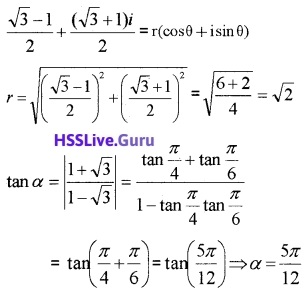The complex number lies in the first quadrant;
⇒ θ = α = $$\frac{5 \pi}{12}$$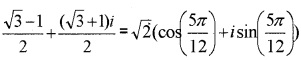Plus One Maths Chapter Wise Questions And Answers Chapter 5 Question 2.

1. Express the complex number $$\frac{2-i}{(1-i)(1+2 i)}$$ in the form a + ib (2)
2. Solve the equation 27x2 – 10x + 1 = 0 (2)

1.2. 27x2 – 10x + 1 = 0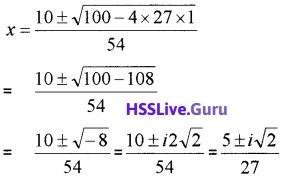Complex Numbers Class 11 Extra Questions Chapter 5  Question 3.

1. For what value of x and y 4x + i(3x – y) = 3 – 6i (2)
2. Solve the equation 21x2 – 28x + 10 = 0 (2)

1. Given; 4x + i(3x – y) = 3 – 6i
⇒ 4x = 3; 3x – y = -6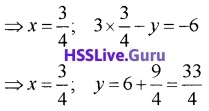2. 21x2 – 28x + 10 = 0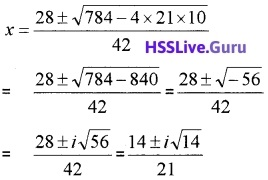Complex Numbers And Quadratic Equations Chapter 5 Question 4.
Consider the complex number z = $$\frac{1+i}{1-i}$$
1. Write z in a + ib form.
2.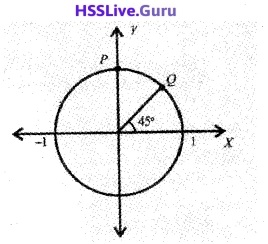In the figure radius of the circle is 1. Write the polar form of the complex number represent by the points P and Q. (2)
3. Find the square root of i. (2)
1.2. Polar form of the point P is $$1\left(\cos \frac{\pi}{2}+i \sin \frac{\pi}{2}\right)$$
Polar form of the point Q is $$1\left(\cos \frac{\pi}{4}+i \sin \frac{\pi}{4}\right)$$

3. i = 0 + i ⇒ $$\sqrt{i}$$ = x + iy ⇒ i = x2 + y2 + 2xyi x2 + y2 = 0; 2xy = 1
(x2 + y2)2 = (x2 – y2)2 + 4x2y2
(x2 + y2)2 = 0 + (1)2 = 1
x2 + y2 = 1; x2 + y2 = 0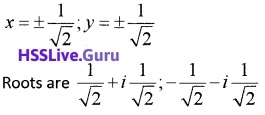### Plus One Maths Complex Numbers and Quadratic Equations Six Mark Questions and Answers

Complex Numbers And Quadratic Equations Class 11 Pdf Question 1.

1. Express the complex number $$\frac{3-\sqrt{-16}}{1-\sqrt{-9}}$$ in the form a + ib (2)
2. Represent the complex number $$\frac{5+i \sqrt{3}}{-4+2 \sqrt{3 i}}$$ in the polar form. (2)
3. Solve the equation ix2 – x + 12i = 0 (2)

1.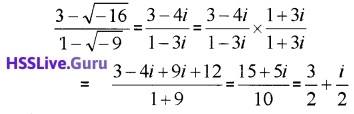2.The complex number lies in the third quadrant;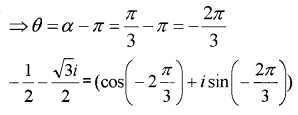3. ix2 – x + 12i = 0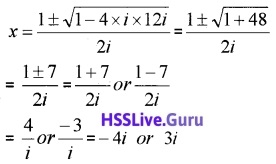### Plus One Maths Complex Numbers and Quadratic Equations Practice Problems Questions and Answers

Complex Numbers And Quadratic Equations Class 11 Solutions Question 1.
Express each of the following in a + ib form. (1 score each)

1. (2 – 4i) + (5 + 3i)
2. (1 – i) – (-1 + 6i)
3. 3(7 + 7i) + i(7 + 7i)
4. $$\left(\frac{1}{5}+i \frac{2}{5}\right)-\left(4+\frac{5}{2} i\right)$$

1. (2 – 4i) + (5 + 3i) = (2 + 5) + (-4 + 3)i = 7 – i

2. (1 – i) – (-1 + 6i) = 1 – i + 1 – 6i = 2 – 7i

3. 3(7 + 7i) + i(7 + 7i) = 21 + 21i + 7i – 7 = 14 + 28i

4.Question 2.
Express each of the following in a + ib form. (1 score each)

1. (-5i)($$\frac{1}{8}$$i)
2. (-i)(2i)(-$$\frac{1}{8}$$i)3
3. i99
4. i111 + i222 + i333
5. (7 – i)(2 + 7i)
6. (-1 – i)(4 + 2i)
7. (5 – 3i)2
8. ($$\frac{1}{3}$$ + 3i)3

1.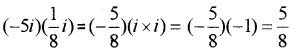2.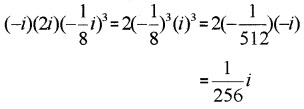3. i99 = i96 + 3 = i96i3 = -i

4. i111 + i222 + i333 + i108 + i220 + 2 + i332 + 1
= i3 + i2 + i1 = -i – 1 + i = -1

5. (7 – i)(2 + 7i) = 7 × 2 – 2i + 7 × 7i – i × 7i
= 14 – 2i + 49i + 7 = 21 + 47i

6. (-1 – i)(4 + 2i) = -4 – 4i – 2i + 2 = – 2 – 6i

7. (5 – 3i)2 = 52 – 2 × 5 × 3i + (3i)2
= 25 – 30i – 9 = 16 – 30i

8.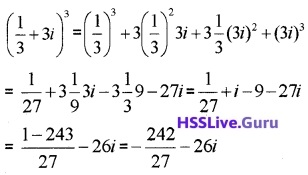Question 3.
Find the multiplicative inverse of the following; (1 score each)

1. 3 – 4i
2. 2 – 3i
3. $$\sqrt{5}$$ + 3i

1. Multiplicative inverse = $$\frac{1}{3-4 i}$$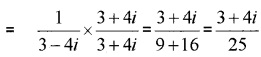2. Multiplicative inverse = $$\frac{1}{2-3 i}$$3. Multiplicative inverse = $$\frac{1}{\sqrt{5}+3 i}$$Question 4.
Express each of the following in a + ib form. (2 score each)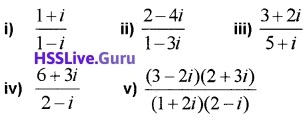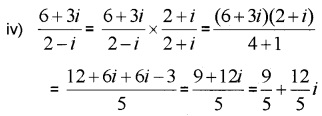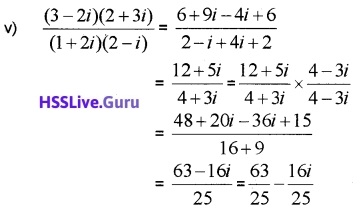Question 5.
Convert the following into polar form. (2 score each)

1. 1 + i
2. -1 + i
3. $$\sqrt{3}$$ – i
4. $$\frac{5-\sqrt{3} i}{4+2 \sqrt{3} i}$$

1. Given; 1 + i = r(cosθ + isinθ)
r = $$\sqrt{1+1}=\sqrt{2}$$
tanα = $$\left|\frac{1}{1}\right|$$ = 1 ⇒ α = $$\frac{\pi}{4}$$
The complex number lies in the first quadrant;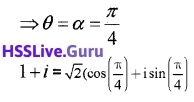2. -1 + i = r(cosθ + isinθ)
r = $$\sqrt{1+1}=\sqrt{2}$$
tanα = $$\left|\frac{1}{-1}\right|$$ = 1 ⇒ α = $$\frac{\pi}{4}$$
The complex number lies in the second quadrant;3.The complex number lies in the fourth quadrant;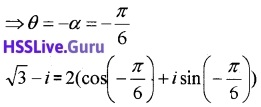4.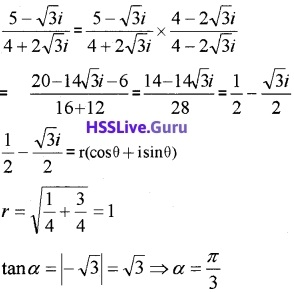The complex number lies in the fourth quadrant;